# Determine the oxidation state of Cl in each species. Identify the oxidation state of Cl in...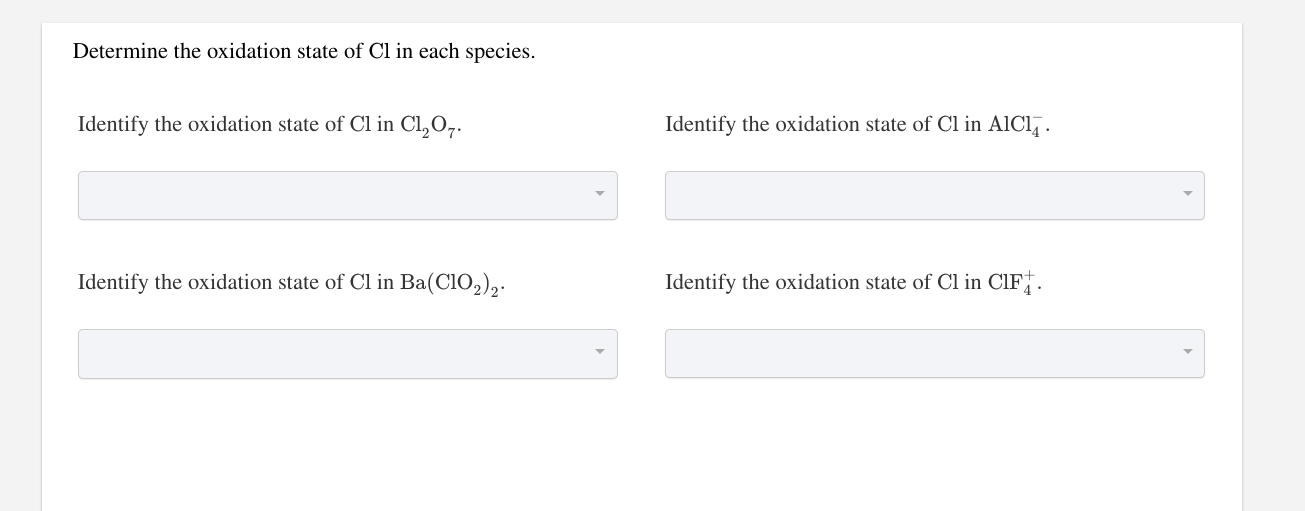Determine the oxidation state of Cl in each species. Identify the oxidation state of Cl in C1,0,. Identify the oxidation state of Cl in AICI. Identify the oxidation state of Cl in Ba(C102), Identify the oxidation state of Cl in CIF..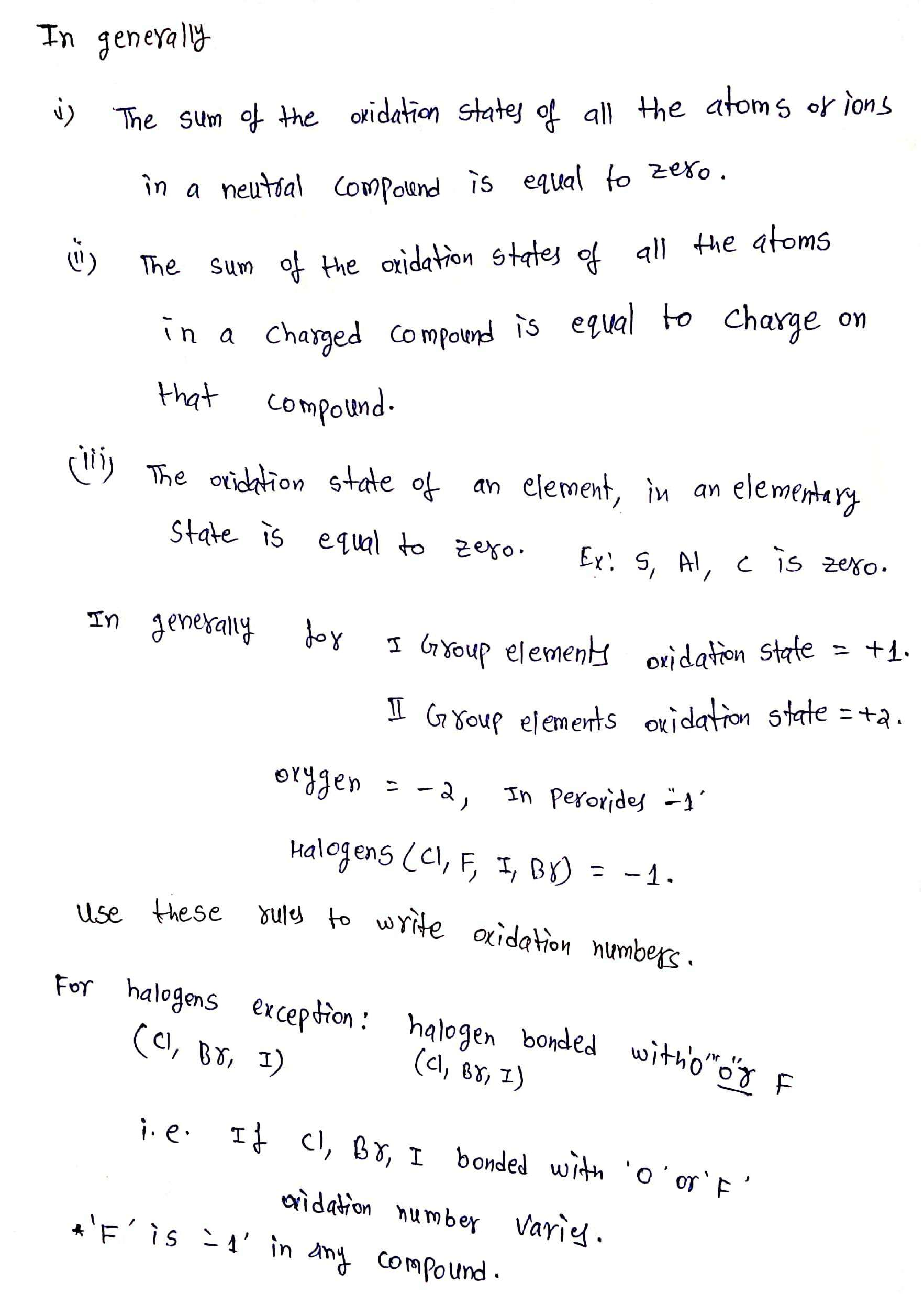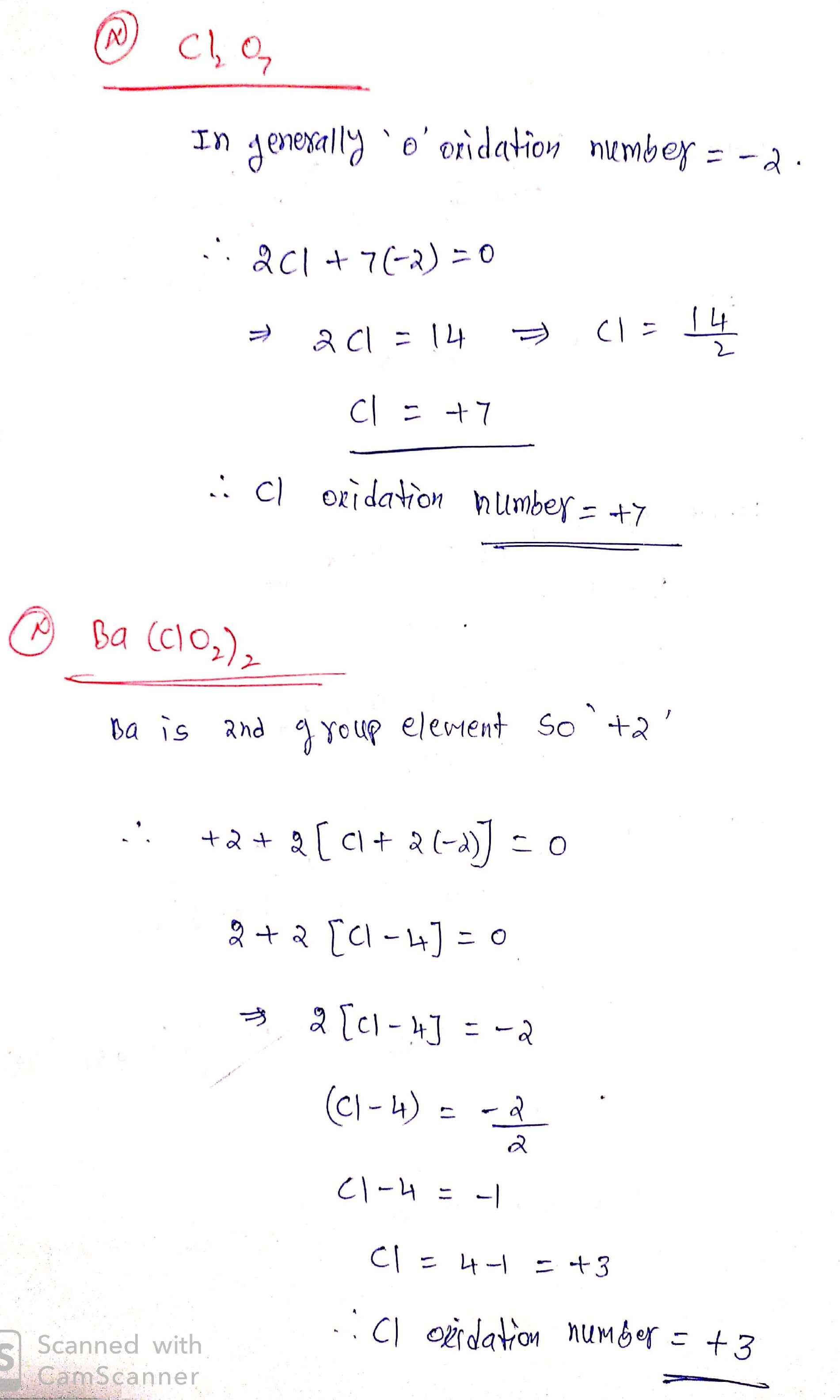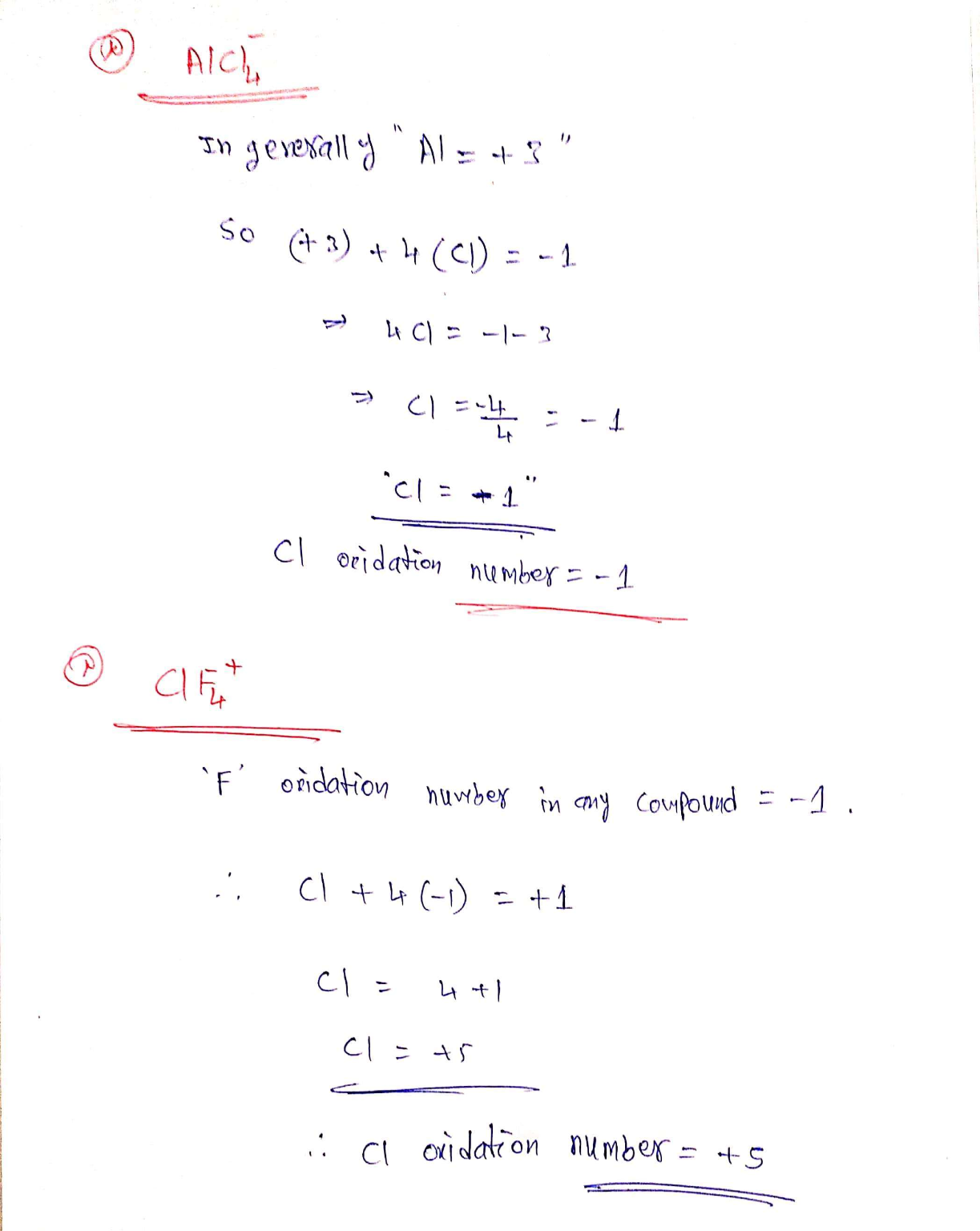#### Earn Coin

Coins can be redeemed for fabulous gifts.

Similar Homework Help Questions
• ### Determine the oxidation state of each species. Identify the oxidation state of Ba2+. Identify the oxidation...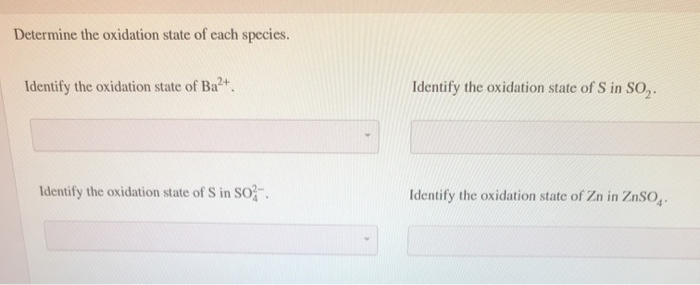Determine the oxidation state of each species. Identify the oxidation state of Ba2+. Identify the oxidation state of Sin SO, Identify the oxidation state of Sin So. Identify the oxidation state of Zn in ZnSO,

• ### oxidation state? most active metal? QUESTION 1 Determine the oxidation state of the following element Sulfur...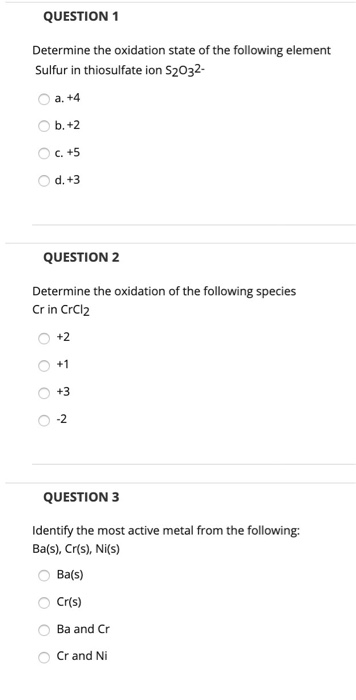oxidation state? most active metal? QUESTION 1 Determine the oxidation state of the following element Sulfur in thiosulfate ion S2032- a. +4 b.+2 C. +5 d. +3 QUESTION 2 Determine the oxidation of the following species Crin CrCl2 +2 0 0 +1 +3 ОО -2 QUESTION 3 Identify the most active metal from the following: Ba(s), Cr(s), Ni(s) Ba(s) Cr(s) Ba and Cr Cr and Ni

• ### 3. Determine the oxidation state for each element. (a) Mn3N2 oxidation state on Mn- Oxidation state...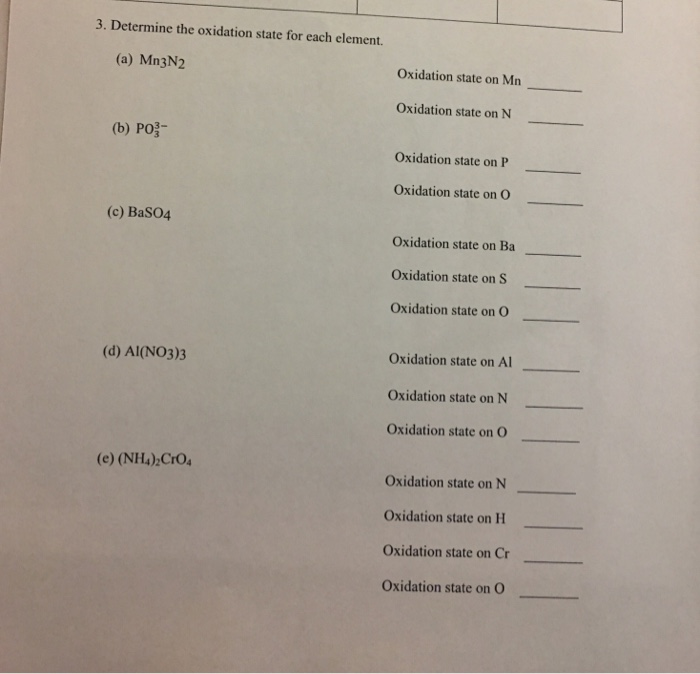3. Determine the oxidation state for each element. (a) Mn3N2 oxidation state on Mn- Oxidation state on N (b) PO- Oxidation state on P Oxidation state on O (c) BaSO4 Oxidation state on Ba Oxidation state on Oxidation state on O Oxidation state on Al Oxidation state on N Oxidation state on O (d) AI(NO3)3 (e) (NH4) CrO Oxidation state on N_ Oxidation state on H Oxidation state on Cr Oxidation state on O

• ### For each of the compounds below, determine the oxidation state of each atom and indicate the...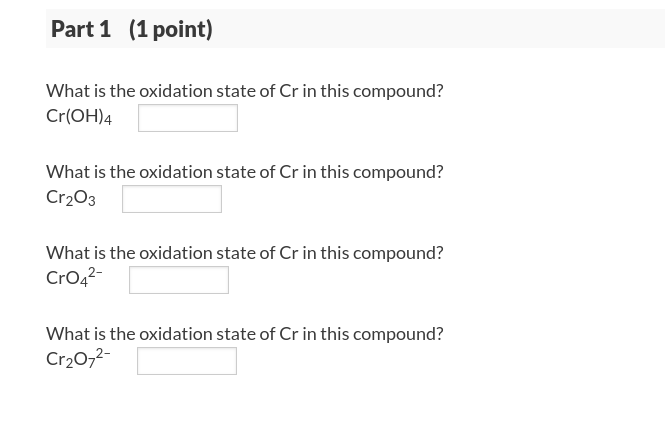For each of the compounds below, determine the oxidation state of each atom and indicate the oxidation states as directed. Part 1 (1 point) What is the oxidation state of Cr in this compound? Cr(OH)4 What is the oxidation state of Cr in this compound? Cr203 What is the oxidation state of Cr in this compound? CrO42 What is the oxidation state of Cr in this compound? Cr20,2 Part 2 (1point) What is the oxidation state of Cl in this...

• ### identify the oxidation state 1. Identify the oxidation state of manganese in each of the following...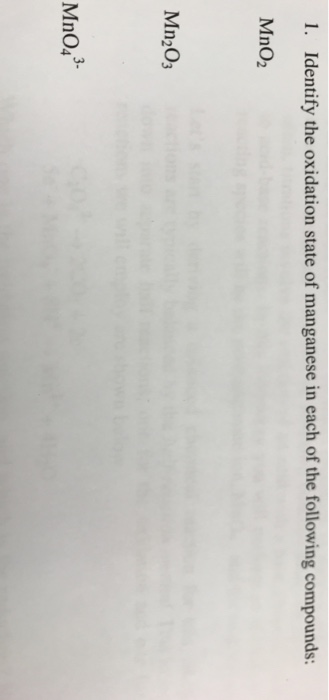identify the oxidation state 1. Identify the oxidation state of manganese in each of the following compounds: MnO2 Mn2O3 3- MnO4

• ### A) B) C) Determine the oxidation state for each of the elements below. iron(?) ion The oxidation state of iron in is...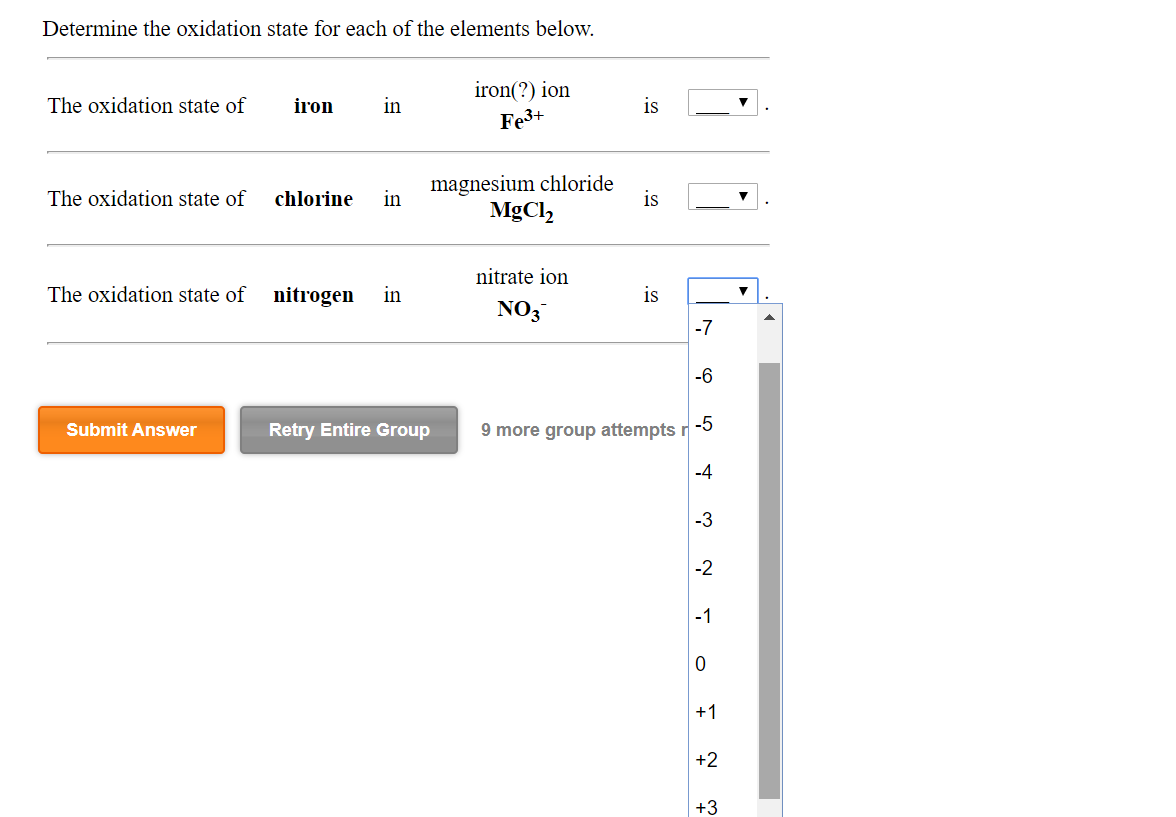A) B) C) Determine the oxidation state for each of the elements below. iron(?) ion The oxidation state of iron in is Fe3+ The oxidation state of chlorine in magnesium chloride MgCl2 is The oxidation state of nitrogen in nitrate ion NO3 is Submit Answer Retry Entire Group 9 more group attempts r-5 Use the rules (in order) to assign oxidation numbers to each of the elements in the compounds below. nitrous acid HNO2 H -_ N _ O _...

• ### next question: Identify the oxidized substance, the reduced substance, the oxidizing agent, and the reducing agent...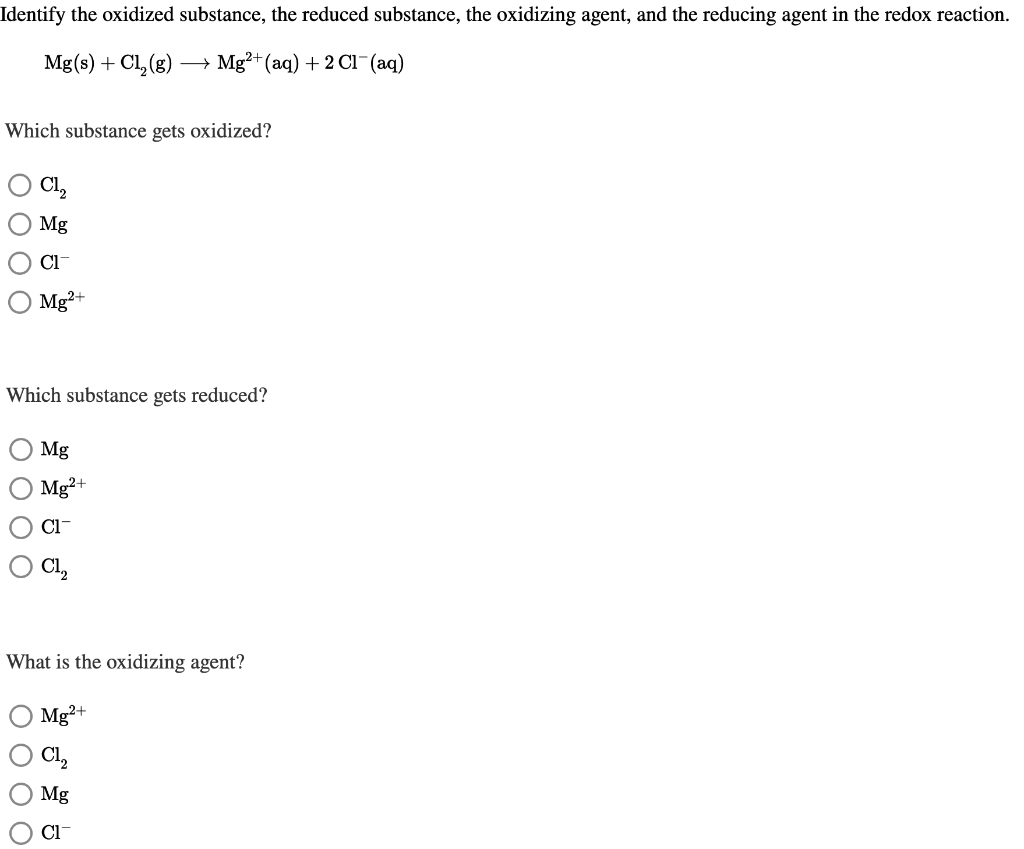next question: Identify the oxidized substance, the reduced substance, the oxidizing agent, and the reducing agent in the redox reaction. Mg(s) + Cl2(g) + Mg2+ (aq) + 2 C1°(aq) Which substance gets oxidized? Oci, Mg OCT Mg2+ Which substance gets reduced? O Mg Mg2+ Ο CI- OCI, What is the oxidizing agent? Mg2+ Oci, OO Mg What is the reducing agent? O cl, Mg2+ Ο CI- Mg Determine the oxidation state of Cl in each species. Identify the oxidation state...

• ### E. Identify the species in the highest oxidation state. : NH NH₂ c =y: HEN А...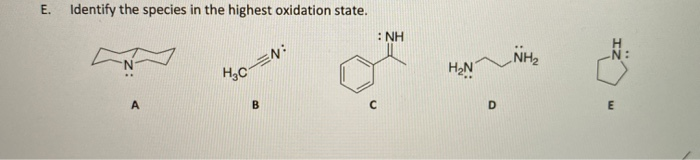E. Identify the species in the highest oxidation state. : NH NH₂ c =y: HEN А D E

• ### 3. Determine the oxidation state for carbon in each species below: C,H, C,H,O 2- CO CO,...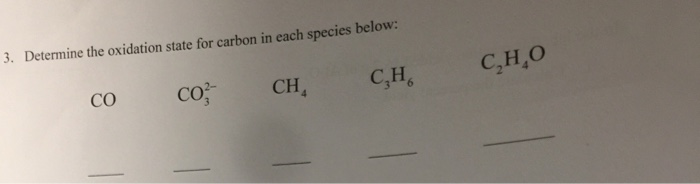3. Determine the oxidation state for carbon in each species below: C,H, C,H,O 2- CO CO, CH

• ### What is the oxidation state of Cl in each of the following ions? Part A ClO-...

What is the oxidation state of Cl in each of the following ions? Part A ClO- Part B ClO2-    Part C ClO3- Part D ClO4-